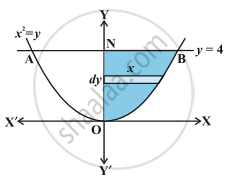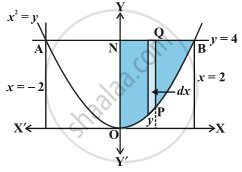Share

# Area of the Region Bounded by a Curve and a Line

#### description

circle-line, elipse-ine, parabola-line

#### notes

We will find the area of the region bounded by a line and a circle, a line and a parabola, a line and an ellipse.Equations of above mentioned curves will be in their standard forms only as the cases in other forms.
For example :
Find the area of the region bounded by the curve y = x2 and the line y = 4.
⇒Since the given curve represented by the equation y = x2 is a parabola symmetrical about y-axis only, therefore, in above  Fig.
The required area of the region AOBA is given by  2int _0^4 xdy =
2("area of the region BONB bounded by curve, y - axis and the lines"
y =0 and y = 4)
= 2 int _0^4 sqrt y  dy = 2 xx 2/3 [y^(3/2)]_0^4 = 4/3 xx  8 = 32/3
Here,we have taken horizontal stripes as indicating in the above Fig.

Alternatively, we may consider the vertical strips like PQ as shown in the following Fig.in fig the area of the region AOBA.  To this end, we solve the equations x2 = y and y = 4 which gives x = –2 and x = 2. Thus, the region AOBA may be stated as the region bounded by the curve y = x2, y = 4 and the ordinates x = –2 and x = 2.
Therefore, the area of the region AOBA
=int _-2^2 y dx
[y=(y-coordinate of Q) - (y-coordinate of P) = 4 - x^2]
= 2 int _0^2(4 - x^2) dx
= 2[4x - x^3/3]_0^2 = 2[4 xx  2 - 8/3 ] = 32 / 3

Remark: From the above examples, it is inferred that we can consider either vertical strips or horizontal strips for calculating the area of the region.

### Shaalaa.com

Application of Integrals part 3 (Area bounded by curve and line) [00:10:01]
S
1
0%